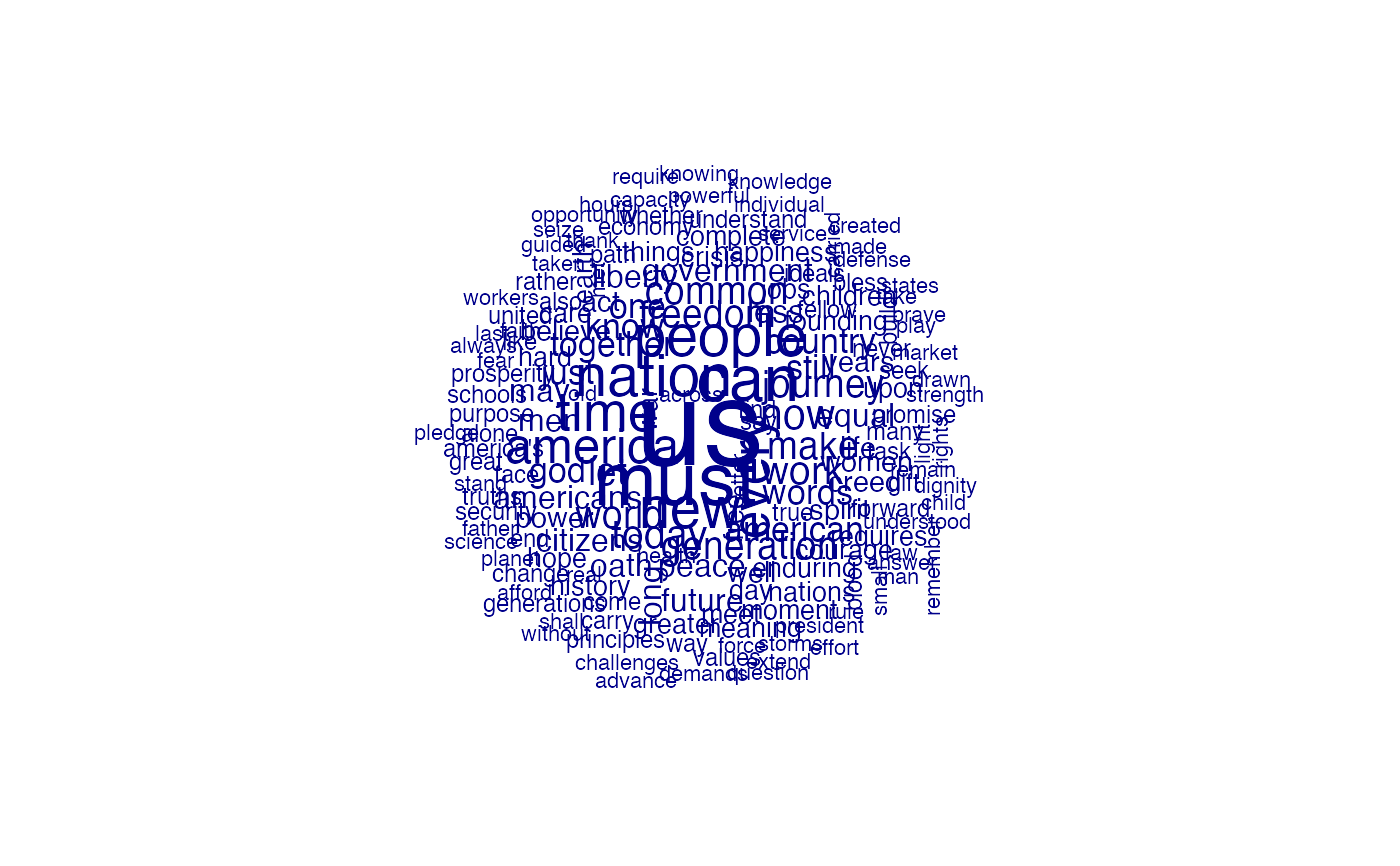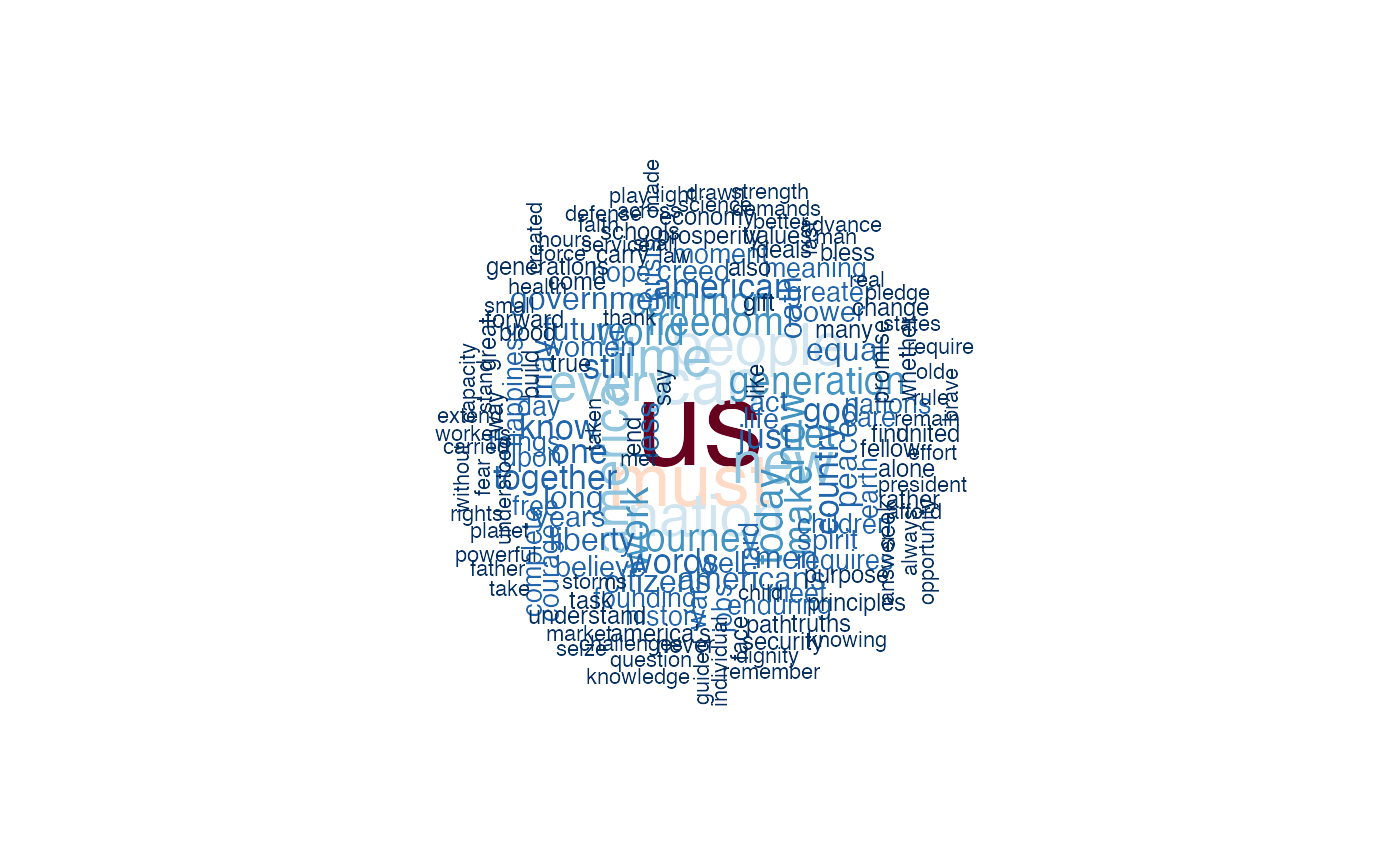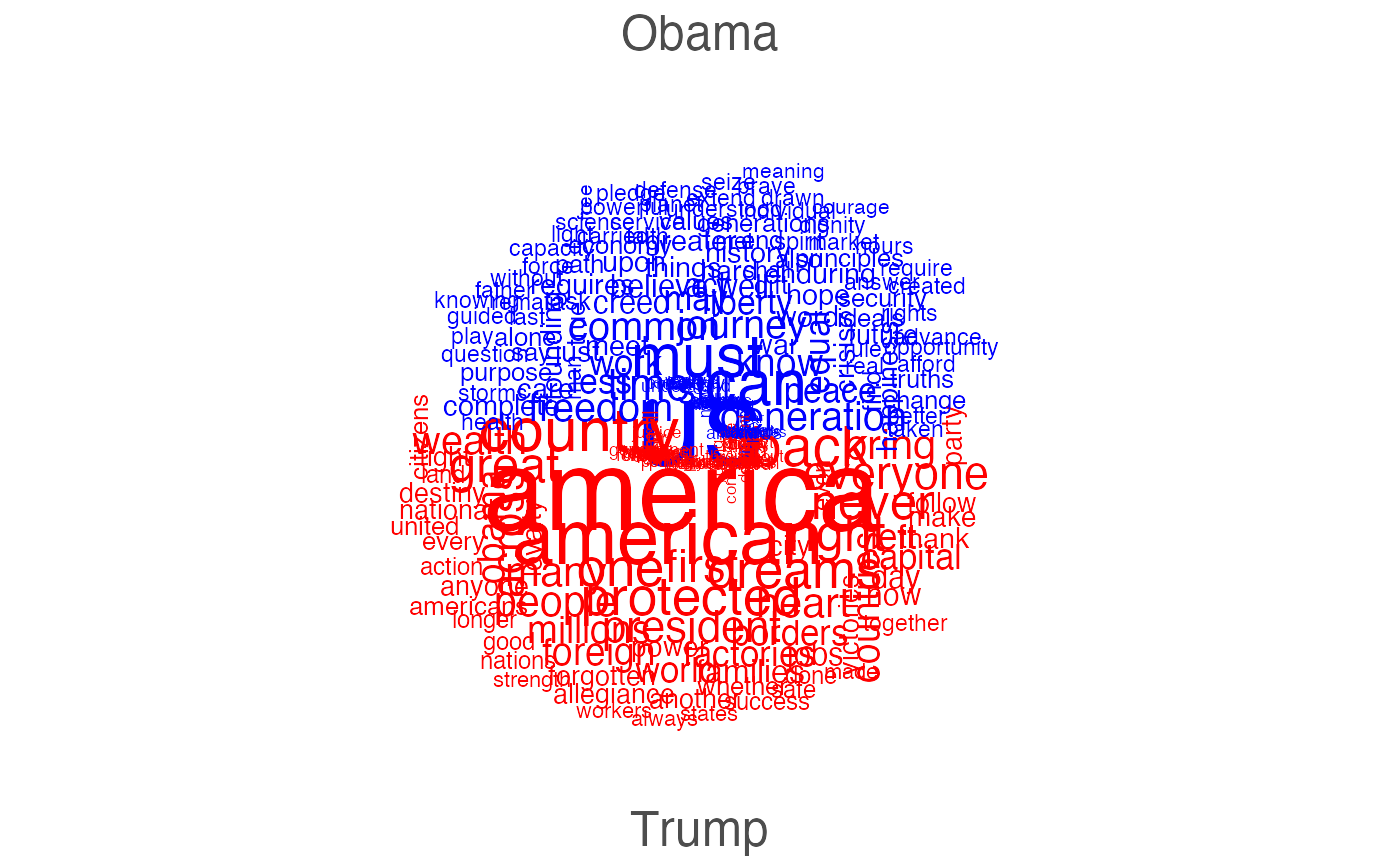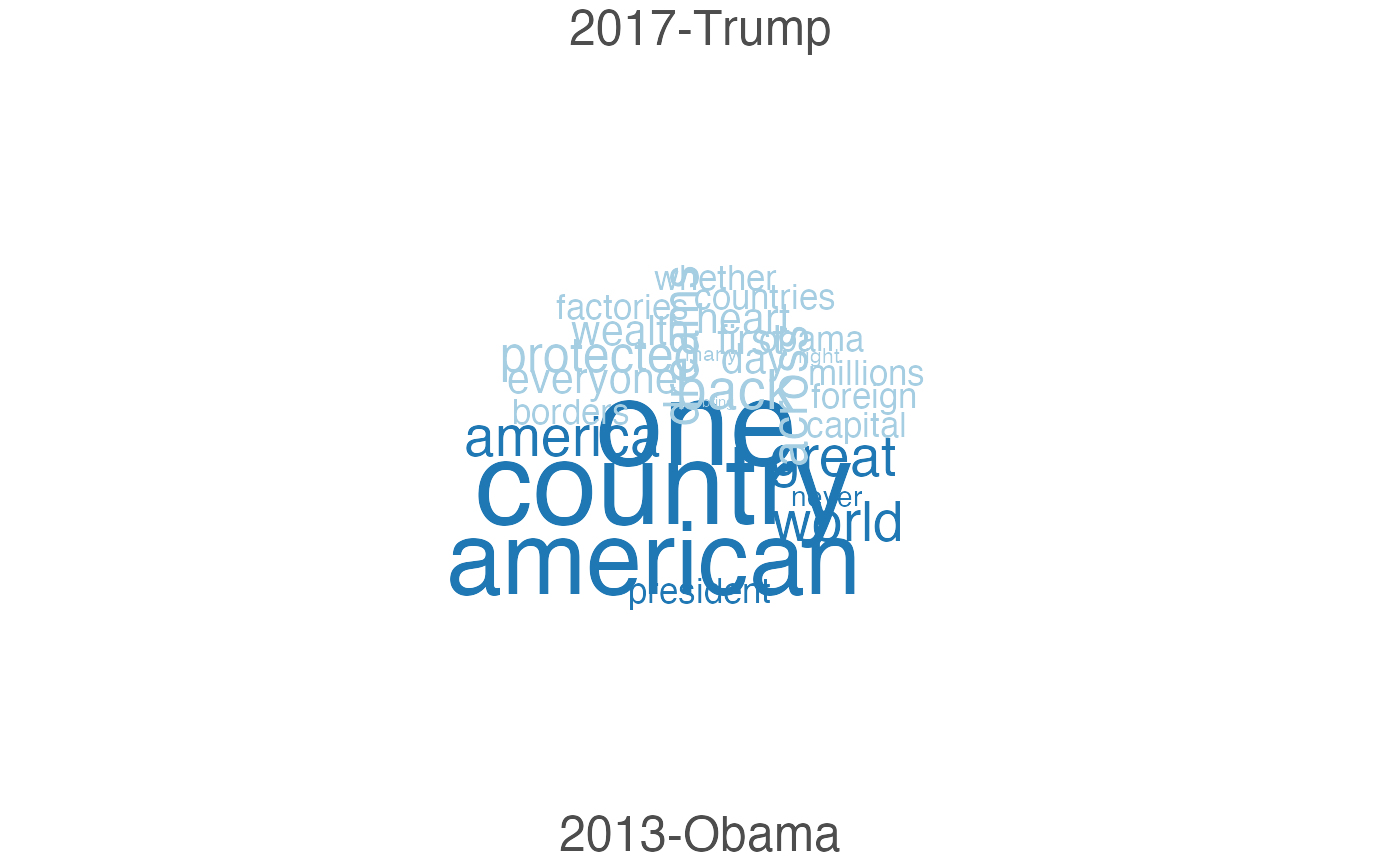Plot a dfm or textstat_keyness object as a wordcloud, where the feature labels are plotted with their sizes proportional to their numerical values in the dfm. When comparison = TRUE, it plots comparison word clouds by document (or by target and reference categories in the case of a keyness object).

textplot_wordcloud(
x,
min_size = 0.5,
max_size = 4,
min_count = 3,
max_words = 500,
color = "darkblue",
font = NULL,
rotation = 0.1,
random_order = FALSE,
random_color = FALSE,
ordered_color = FALSE,
labelcolor = "gray20",
labelsize = 1.5,
labeloffset = 0,
fixed_aspect = TRUE,
...,
comparison = FALSE
)

## Arguments

x a dfm or textstat_keyness object size of the smallest word size of the largest word words with frequency below min_count will not be plotted maximum number of words to be plotted. The least frequent terms dropped. The maximum frequency will be split evenly across categories when comparison = TRUE. color of words from least to most frequent font-family of words and labels. Use default font if NULL. adjust sizes of words by a constant. Useful for non-English words for which R fails to obtain correct sizes. proportion of words with 90 degree rotation plot words in random order. If FALSE, they will be plotted in decreasing frequency. choose colors randomly from the colors. If FALSE, the color is chosen based on the frequency if TRUE, then colors are assigned to words in order. color of group labels. Only used when comparison = TRUE. size of group labels. Only used when comparison = TRUE. position of group labels. Only used when comparison = TRUE. logical; if TRUE, the aspect ratio is fixed. Variable aspect ratio only supported if rotation = 0. additional parameters. Only used to make it compatible with wordcloud logical; if TRUE, plot a wordcloud that compares documents in the same way as wordcloud::comparison.cloud(). If x is a textstat_keyness object, then only the target category's key terms are plotted when comparison = FALSE, otherwise the top max_words / 2 terms are plotted from the target and reference categories.

## Details

The default is to plot the word cloud of all features, summed across documents. To produce word cloud plots for specific document or set of documents, you need to slice out the document(s) from the dfm object.

Comparison wordcloud plots may be plotted by setting comparison = TRUE, which plots a separate grouping for each document in the dfm. This means that you will need to slice out just a few documents from the dfm, or to create a dfm where the "documents" represent a subset or a grouping of documents by some document variable.

## Author

Kohei Watanabe, building on code from Ian Fellows's wordcloud package.

## Examples

# plot the features (without stopwords) from Obama's inaugural addresses
set.seed(10)
dfmat1 <- dfm(corpus_subset(data_corpus_inaugural, President == "Obama"),
remove = stopwords("english"), remove_punct = TRUE) %>%
dfm_trim(min_termfreq = 3)

# basic wordcloud
textplot_wordcloud(dfmat1)# plot in colors with some additional options
textplot_wordcloud(dfmat1, rotation = 0.25,
color = rev(RColorBrewer::brewer.pal(10, "RdBu")))# other display options
col <- sapply(seq(0.1, 1, 0.1), function(x) adjustcolor("#1F78B4", x))
textplot_wordcloud(dfmat1, adjust = 0.5, random_order = FALSE,
color = col, rotation = FALSE)# comparison plot of Obama v. Trump
dfmat2 <- dfm(corpus_subset(data_corpus_inaugural, President %in% c("Obama", "Trump")),
remove = stopwords("english"), remove_punct = TRUE, groups = "President") %>%
dfm_trim(min_termfreq = 3)

textplot_wordcloud(dfmat2, comparison = TRUE, max_words = 300,
color = c("blue", "red"))# for keyness
tstat <- tail(data_corpus_inaugural, 2) %>%
dfm(remove_punct = TRUE, remove = stopwords("en")) %>%
textstat_keyness(target = 2)
textplot_wordcloud(tstat, max_words = 100)textplot_wordcloud(tstat, comparison = FALSE, max_words = 100)